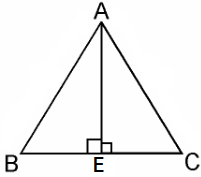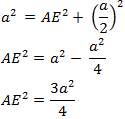Guru

# In an equilateral triangle, prove that three times the square of one side is equal to four times the square of one of its altitudes. Q.16

• 0

What is the best way to solve the problem of ncert question of exercise 6.5 of triangles chapter for class 10th How i solve this problem in easy and simple way In an equilateral triangle, prove that three times the square of one side is equal to four times the square of one of its altitudes.

Share

1. Given, an equilateral triangle say ABC,Let the sides of the equilateral triangle be of length a, and AE be the altitude of ΔABC.

∴ BE = EC = BC/2 = a/2

In ΔABE, by Pythagoras Theorem, we get

AB2 = AE2 + BE24AE2 = 3a2

⇒ 4 × (Square of altitude) = 3 × (Square of one side)

Hence, proved.

• 0